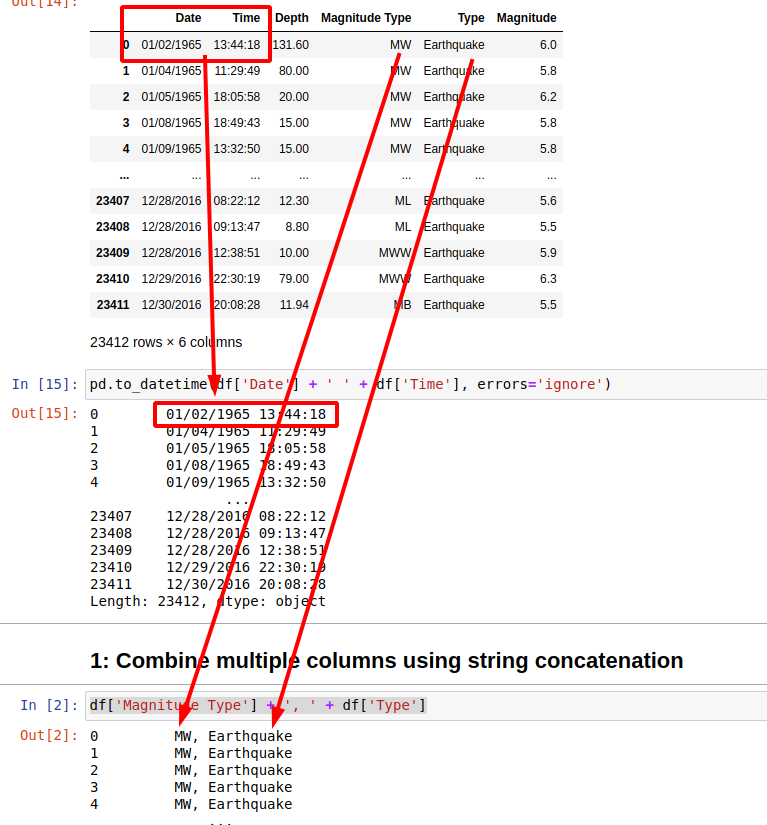In this short guide, you'll see how to combine multiple columns into a single one in Pandas.

Here you can find the short answer:

(1) String concatenation

``````df['Magnitude Type'] + ', ' + df['Type']
``````

(2) Using methods `agg` and `join`

``````df[['Date', 'Time']].T.agg(','.join)
``````

(3) Using lambda and join

``````df[['Date', 'Time']].agg(lambda x: ','.join(x.values), axis=1).T
``````

So let's see several useful examples on how to combine several columns into one with Pandas.

Suppose you have data like:

Date Time Depth Magnitude Type Type Magnitude
01/02/1965 13:44:18 131.6 MW Earthquake 6.0
01/04/1965 11:29:49 80.0 MW Earthquake 5.8
01/05/1965 18:05:58 20.0 MW Earthquake 6.2
01/08/1965 18:49:43 15.0 MW Earthquake 5.8
01/09/1965 13:32:50 15.0 MW Earthquake 5.8

## 1: Combine multiple columns using string concatenation

Let's start with most simple example - to combine two string columns into a single one separated by a comma:

``````df['Magnitude Type'] + ', ' + df['Type']
``````

result will be:

``````0         MW, Earthquake
1         MW, Earthquake
2         MW, Earthquake
3         MW, Earthquake
4         MW, Earthquake
``````

What if one of the columns is not a string? Then you will get error like:

TypeError: can only concatenate str (not "float") to str

To avoid this error you can convert the column by using method `.astype(str)`:

``````df['Magnitude Type'] + ', ' + df['Magnitude'].astype(str)
``````

result:

``````0         MW, 6.0
1         MW, 5.8
2         MW, 6.2
3         MW, 5.8
4         MW, 5.8
``````

## 2: Combine date and time columns into DateTime column

What if you have separate columns for the date and the time. You can concatenate them into a single one by using string concatenation and conversion to datetime:

``````pd.to_datetime(df['Date'] + ' ' + df['Time'], errors='ignore')
``````

In case of missing or incorrect data we will need to add parameter: `errors='ignore'` in order to avoid error:

ParserError: Unknown string format: 1975-02-23T02:58:41.000Z 1975-02-23T02:58:41.000Z## 3: Combine multiple columns with agg and join

Another option to concatenate multiple columns is by using two Pandas methods:

• `agg`
• `join`
``````df[['Date', 'Time']].T.agg(','.join)
``````

result:

``````0        01/02/1965,13:44:18
1        01/04/1965,11:29:49
2        01/05/1965,18:05:58
3        01/08/1965,18:49:43
``````

This one might be a bit slower than the first one.

## 4: Combine multiple columns with lambda and join

You can use lambda expressions in order to concatenate multiple columns. The advantages of this method are several:

• you can have condition on your input - like filter
• output can be customised
• better control on dtypes

To combine columns date and time we can do:

``````df[['Date', 'Time']].agg(lambda x: ','.join(x.values), axis=1).T
``````

In the next section you can find how we can use this option in order to combine columns with the same name.

## 5: Combine columns which have the same name

Finally let's combine all columns which have exactly the same name in a Pandas DataFrame.

First let's create duplicate columns by:

``````df.columns = ['Date', 'Date', 'Depth', 'Magnitude Type', 'Type', 'Magnitude']
df
``````

A general solution which concatenates columns with duplicate names can be:

``````df.groupby(df.columns, axis=1).agg(lambda x: x.apply(lambda y: ','.join([str(l) for l in y if str(l) != "nan"]), axis=1))
``````

This will result into:

Date Depth Magnitude Magnitude Type Type
01/02/1965,13:44:18 131.6 6.0 MW Earthquake
01/04/1965,11:29:49 80.0 5.8 MW Earthquake
01/05/1965,18:05:58 20.0 6.2 MW Earthquake
01/08/1965,18:49:43 15.0 5.8 MW Earthquake
01/09/1965,13:32:50 15.0 5.8 MW Earthquake

How does it work? First is grouping the columns which share the same name:

``````for i in df.groupby(df.columns, axis=1):
print(i)
``````

result:

``````('Date',              Date      Date
0      01/02/1965  13:44:18
1      01/04/1965  11:29:49
2      01/05/1965  18:05:58
3      01/08/1965  18:49:43
4      01/09/1965  13:32:50
...           ...       ...
23407  12/28/2016  08:22:12
23408  12/28/2016  09:13:47
23409  12/28/2016  12:38:51
23410  12/29/2016  22:30:19
23411  12/30/2016  20:08:28

[23412 rows x 2 columns])
('Depth',         Depth
0      131.60
1       80.00
2       20.00
3       15.00
4       15.00
...       ...
23407   12.30
23408    8.80
``````

Then it's combining their values:

``````df.groupby(df.columns, axis=1).apply(lambda x: x.values)
``````

result:

``````Date              [[01/02/1965, 13:44:18], [01/04/1965, 11:29:49...
Depth             [[131.6], [80.0], [20.0], [15.0], [15.0], [35....
Magnitude         [[6.0], [5.8], [6.2], [5.8], [5.8], [6.7], [5....
Magnitude Type    [[MW], [MW], [MW], [MW], [MW], [MW], [MW], [MW...
Type              [[Earthquake], [Earthquake], [Earthquake], [Ea...
``````

Finally there is prevention of errors in case of bad values like NaN, missing values, None, different formats etc.